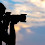# Facebook style time ago function using PHP

In this tutorial we are going to learn how to make Facebook style time ago function in PHP. Friends If you have used Facebook and you update status on your wall or you upload photo on your Facebook wall then after uploading it display time as Just Now or you can also see your previous post time, it show like one week or month or year or day. In this tutorial we will learn how to come this type of time using php programming language. This is a very simple php script of get output like Facebook style time ago. In this function we can calculate time using particular country timezone. If you have previous date and time then in this function it count time between current date and time to this previous date time on simple php logic. In this function I convert previous date and time to UNIX time stamp and current date and time also get in UNIX time. From difference of this current UNIX time stamp and previous date and time time stamp we can get number of seconds between these date and time. From seconds we can easily get minutes, hours, days, weeks, months and years. In this post I also provide source code with this post.

## Source Code

`````` <?php
date_default_timezone_set('America/New_York');
{
\$time_ago = strtotime(\$timestamp);
\$current_time = time();
\$time_difference = \$current_time - \$time_ago;
\$seconds = \$time_difference;
\$minutes      = round(\$seconds / 60 );           // value 60 is seconds
\$hours           = round(\$seconds / 3600);           //value 3600 is 60 minutes * 60 sec
\$days          = round(\$seconds / 86400);          //86400 = 24 * 60 * 60;
\$weeks          = round(\$seconds / 604800);          // 7*24*60*60;
\$months          = round(\$seconds / 2629440);     //((365+365+365+365+366)/5/12)*24*60*60
\$years          = round(\$seconds / 31553280);     //(365+365+365+365+366)/5 * 24 * 60 * 60
if(\$seconds <= 60)
{
return "Just Now";
}
else if(\$minutes <=60)
{
if(\$minutes==1)
{
return "one minute ago";
}
else
{
return "\$minutes minutes ago";
}
}
else if(\$hours <=24)
{
if(\$hours==1)
{
return "an hour ago";
}
else
{
return "\$hours hrs ago";
}
}
else if(\$days <= 7)
{
if(\$days==1)
{
return "yesterday";
}
else
{
return "\$days days ago";
}
}
else if(\$weeks <= 4.3) //4.3 == 52/12
{
if(\$weeks==1)
{
return "a week ago";
}
else
{
return "\$weeks weeks ago";
}
}
else if(\$months <=12)
{
if(\$months==1)
{
return "a month ago";
}
else
{
return "\$months months ago";
}
}
else
{
if(\$years==1)
{
return "one year ago";
}
else
{
return "\$years years ago";
}
}
}
?>
``````

1.Hy!
Thank you so much for giving us such a nice illustration of php basics as well as expert level concepts.
I was in search of such a video tutorials that help us to learn the php according to latest standards.
..
Carry on !
God bless you

2.Thank Sir... ANG GALING

3.my date comes from mysql php echo. how do i implement this?

4.My date cames from mysql php echo. how do i implement this?

5.6.Not working properly on a live server, always starting from 7hours ago on every post

7.Thanks you so much sir

8.Not working on localhost.showing Just Now in every fucking situation.

9.thank you sir

10.not working properly on live server.
I have chatting system in which users have different timezone.
And it is not working as expected.

11.works perfectly .

12.